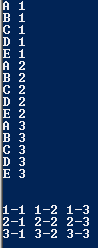# Assignemnt #111

## Code

```//colin hinton
//nest.java
//5/4/2016
//5th period

public class nest
{
public static void main( String[] args )
{
// this is #1 - I'll call it "CN"
for ( int n=1; n <= 3; n++ )
{
for ( char c='A'; c <= 'E'; c++ ) //The inner one changes faster and is controlled by the innter loop
{
System.out.println( c + " " + n );
}
}
//swapping the two puts numbers before letters
System.out.println("\n");

// this is #2 - I'll call it "AB"//with println they now print each line
for ( int a=1; a <= 3; a++ )
{
for ( int b=1; b <= 3; b++ )
{
System.out.print( a + "-" + b + " " );
}
System.out.println();//output now has space from lines.
}

System.out.println("\n");

}
}
Picture of the output```